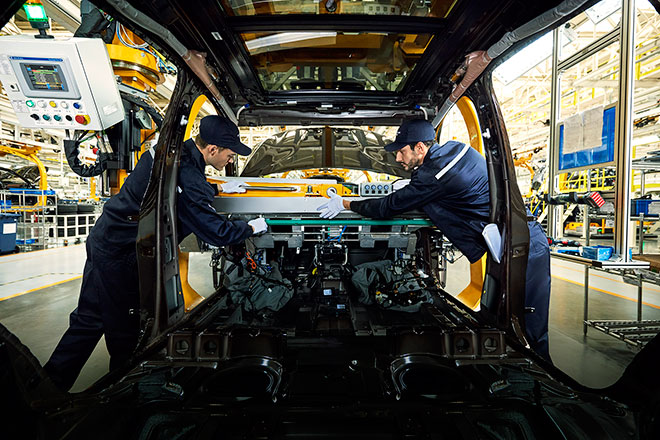匠心诠释豪华品质 走进宝沃汽车工业4.0智能工厂

2018年03月29日 14:53 来源：车行天下 超过：13739次关注

1919年，Carl Friedrich Wilhelm Borgward（卡尔·宝沃）在德国不莱梅创建宝沃品牌，其以革命性的技术、引领风潮的设计，全面的产品谱系、超过当时德国60%的出口份额，一度成为德国第三大汽车生产制造商。2016年宝沃汽车携带新品BX7正式进入中国，作为进军中国汽车市场的一个“全新”品牌，难免会有行业内的质疑，但是宝沃汽车迅速用实力征服了市场与消费者，凭借卓越的产品品质、优质的售后服务，已经得到市场的高度认可。如今其产品线已经拥有三款高品质的量产车型，分别是BX5、BX7和BX7 TS，近期小编有幸领略了一番宝沃汽车的生产厂商并试驾了其当下的三款产品，现就让小编带大家详细介绍一下本次宝沃工厂的参观之旅。BORGWARD，包含“BORG”和“WARD”两层含义，寓意“城堡守护者”，充满品牌魅力与时代张力。官方表示：“新Logo精致的棱角变化体现‘不拘一格，突破进化’，以暗藏的细腻纹理体现‘千泽万钻，合力共创’，以局部镂空的设计体现‘自由思想，拥抱未来’。具有张力的设计风格，赋予了Borgward品牌新的精神内涵和饱满的生命力。拥有全球首条八车型智能生产系统的宝沃工业4.0智能工厂

“柔”、“精”、”严”，是宝沃智能工厂的三大生产核心理念。其中，全球首条8种不同车型柔性生产线将生产效率最大化，3分钟之内即可完成整线自动切换模式，而拥有222套柔性NC机器人定位系统还可满足同一条生产线不同车身的定位；加之17套集中颜色供应系统，更能快速满足客户定制需求。同时，在对生产精益求精的追求下，宝沃智能工厂凭借超过5,000个车身焊点数领先行业标准，而误差不超过0.3毫米的零件精度、22,000多个整车测量点和超过290种内饰配件的精细匹配，能够保证车辆从内至外的精致美观。此外，滴水不漏的严苛普检更拥有国际领先的3种全项目检测线，1,075个整车检测项目更对车辆质量问题严防死守，而在14种100%全路谱道路测试和360度无死角淋雨检测下，车辆将经历魔鬼般的严苛测试才能顺利出厂。正是在这样高标准的智造理念下，每一辆宝沃汽车才能以全方位的德国高品质标准，交付至每一位车主手中。相关文章

0-500 字已有评论 0条 查看评论>>

﻿
• 快速找车
• 选择品牌
• 选择品牌
• A  奥迪
• A  阿斯顿·马丁
• A  阿尔法·罗密欧
• B  宝沃
• B  布加迪
• B  巴博斯
• B  保时捷
• B  宾利
• B  奔驰
• B  宝马
• B  本田
• B  别克
• B  标致
• B  比亚迪
• B  宝骏
• B  北汽制造
• B  北汽新能源
• B  北汽幻速
• B  北汽威旺
• B  北京汽车
• B  奔腾
• B  北汽绅宝
• C  长安
• C  长安商用
• C  长城
• C  昌河
• D  大众
• D  道奇
• D  DS
• D  东南
• D  东风风神
• D  东风风行
• D  东风小康
• D  东风风度
• D  东风
• F  福特
• F  丰田
• F  菲亚特
• F  法拉利
• F  福田
• F  福迪
• F  福汽启腾
• G  观致
• G  广汽传祺
• G  广汽吉奥
• G  GMC
• H  红旗
• H  汉腾汽车
• H  哈弗
• H  哈飞
• H  海格
• H  海马
• H  华颂
• H  黄海
• H  华泰
• H  恒天
• J  吉利汽车
• J  捷豹
• J  Jeep
• J  江淮
• J  江铃
• J  金杯
• J  九龙
• J  金旅
• K  凯翼
• K  凯迪拉克
• K  克莱斯勒
• K  科尼塞克
• K  卡威
• K  开瑞
• L  路虎
• L  林肯
• L  劳斯莱斯
• L  兰博基尼
• L  雷克萨斯
• L  铃木
• L  雷诺
• L  理念
• L  力帆
• L  莲花汽车
• L  猎豹
• L  路特斯
• L  陆风
• M  马自达
• M  MG
• M  MINI
• M  玛莎拉蒂
• M  摩根
• M  迈凯轮
• N  纳智捷
• O  欧宝
• O  讴歌
• O  欧朗
• Q  奇瑞
• Q  起亚
• Q  启辰
• R  日产
• R  荣威
• R  瑞麒
• S  三菱
• S  斯威汽车
• S  萨博
• S  smart
• S  斯柯达
• S  斯巴鲁
• S  思铭
• S  双龙
• S  上汽大通
• S  双环
• T  特斯拉
• T  腾势
• W  沃尔沃
• W  五菱汽车
• W  五十铃
• W  威兹曼
• W  威麟
• X  现代
• X  雪佛兰
• X  雪铁龙
• X  西雅特
• Y  一汽
• Y  英菲尼迪
• Y  英致
• Y  依维柯
• Y  野马汽车
• Y  永源
• Z  众泰
• Z  中华
• Z  中兴
• Z  知豆
• 选择车系
• 选择车系
• 车型对比
• 选择品牌
• 选择品牌
• A  奥迪
• A  阿斯顿·马丁
• A  阿尔法·罗密欧
• B  宝沃
• B  布加迪
• B  巴博斯
• B  保时捷
• B  宾利
• B  奔驰
• B  宝马
• B  本田
• B  别克
• B  标致
• B  比亚迪
• B  宝骏
• B  北汽制造
• B  北汽新能源
• B  北汽幻速
• B  北汽威旺
• B  北京汽车
• B  奔腾
• B  北汽绅宝
• C  长安
• C  长安商用
• C  长城
• C  昌河
• D  大众
• D  道奇
• D  DS
• D  东南
• D  东风风神
• D  东风风行
• D  东风小康
• D  东风风度
• D  东风
• F  福特
• F  丰田
• F  菲亚特
• F  法拉利
• F  福田
• F  福迪
• F  福汽启腾
• G  观致
• G  广汽传祺
• G  广汽吉奥
• G  GMC
• H  红旗
• H  汉腾汽车
• H  哈弗
• H  哈飞
• H  海格
• H  海马
• H  华颂
• H  黄海
• H  华泰
• H  恒天
• J  吉利汽车
• J  捷豹
• J  Jeep
• J  江淮
• J  江铃
• J  金杯
• J  九龙
• J  金旅
• K  凯翼
• K  凯迪拉克
• K  克莱斯勒
• K  科尼塞克
• K  卡威
• K  开瑞
• L  路虎
• L  林肯
• L  劳斯莱斯
• L  兰博基尼
• L  雷克萨斯
• L  铃木
• L  雷诺
• L  理念
• L  力帆
• L  莲花汽车
• L  猎豹
• L  路特斯
• L  陆风
• M  马自达
• M  MG
• M  MINI
• M  玛莎拉蒂
• M  摩根
• M  迈凯轮
• N  纳智捷
• O  欧宝
• O  讴歌
• O  欧朗
• Q  奇瑞
• Q  起亚
• Q  启辰
• R  日产
• R  荣威
• R  瑞麒
• S  三菱
• S  斯威汽车
• S  萨博
• S  smart
• S  斯柯达
• S  斯巴鲁
• S  思铭
• S  双龙
• S  上汽大通
• S  双环
• T  特斯拉
• T  腾势
• W  沃尔沃
• W  五菱汽车
• W  五十铃
• W  威兹曼
• W  威麟
• X  现代
• X  雪佛兰
• X  雪铁龙
• X  西雅特
• Y  一汽
• Y  英菲尼迪
• Y  英致
• Y  依维柯
• Y  野马汽车
• Y  永源
• Z  众泰
• Z  中华
• Z  中兴
• Z  知豆
• 选择车系
• 选择车系
• 选择车型
• 选择车型
• 意见反馈